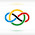### 1970 IMO SL #10

The real numbers $a_0,a_1,a_2,\ldots$ satisfy $1=a_0\le a_1\le a_2\le\ldots. b_1,b_2,b_3,\ldots$ are defined by $b_n=\sum_{k=1}^n{1-{a_{k-1}\over a_k}\over\sqrt a_k}$.

a) Prove that $0\le b_n<2$.

b) Given $c$ satisfying $0\le c<2$, prove that we can find $a_n$ so that $b_n>c$ for all sufficiently large $n$.

a) We claim that $$\frac{1 - \frac{a_{k - 1}}{a_{k}}}{\sqrt{a_{k}}} \leqslant 2\left(\frac{1}{\sqrt{a_{k - 1}}} - \frac{1}{\sqrt{a_{k}}}\right).$$ By AM-GM on $\frac{a_{k - 1}}{a_{k}^{\frac{3}{2}}}, \frac{1}{\sqrt{a_{k - 1}}}, \frac{1}{\sqrt{a_{k - 1}}}$ we get$\frac{a_{k - 1}}{a_{k}^{\frac{3}{2}}} + \frac{2}{\sqrt{a_{k - 1}}} \geqslant \frac{3}{\sqrt{a_{k}}},$ or $\frac{1 - \frac{a_{k - 1}}{a_{k}}}{\sqrt{a_{k}}} = \frac{1}{\sqrt{a_{k}}} - \frac{a_{k - 1}}{a_{k}^{\frac{3}{2}}} \leqslant \frac{2}{\sqrt{a_{k - 1}}} - \frac{2}{\sqrt{a_{k}}} = 2\left(\frac{1}{\sqrt{a_{k - 1}}} - \frac{1}{\sqrt{a_{k}}}\right),$as required. Hence, $$b_{n} = \sum_{k = 1}^{n} \frac{1 - \frac{a_{k - 1}}{a_{k}}}{\sqrt{a_{k}}} \leqslant \sum_{k = 1}^{n} 2\left(\frac{1}{\sqrt{a_{k - 1}}} - \frac{1}{\sqrt{a_{k}}}\right) = 2\left(\frac{1}{\sqrt{a_{0}}} - \frac{1}{\sqrt{a_{n}}}\right) < \frac{2}{\sqrt{a_{0}}} = 2,$$ as desired by our claim. Now, summing this over $k=1, \cdots, n$, we obtain $$b_n \leq 2\left(\frac{1}{\sqrt{a_0}}-\frac{1}{\sqrt{a_n}}\right ) < 2.$$ The desired then follows. $\square$

b) Consider $a_k=q^{-2k}$ where $0<q<1$. Then, $$b_n=q(1+q)(1-q^n)>2q^2(1-q^n)$$. Next, consider $n>\log_q(1-q)$. Then we have $b_n>2q^3$. Now taking $q\ge\sqrt{\frac{c}{2}}$, we obtain $b_n>c$, as desired. $\square$

1.Anonymous2/01/2022

oohh new post :)

the first thing i check in the morning is your blog to look for a new post :)

2.Anonymous2/01/2022

@above me too lol

did you happen to post this late night (EST) yesterday?

i checked several times yesterday for a new post but i didnt see this

3.@anonymous (comment #2)

Yes, I did post this near 11:00 PM EST.